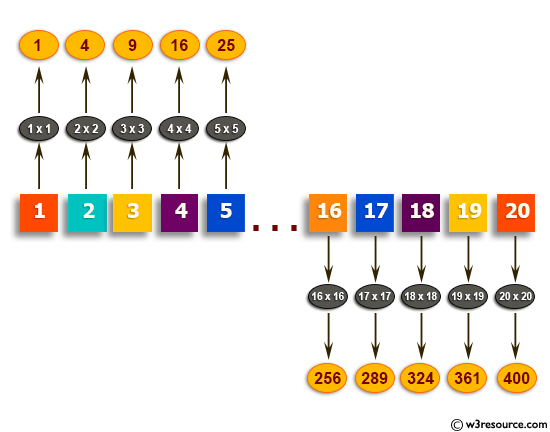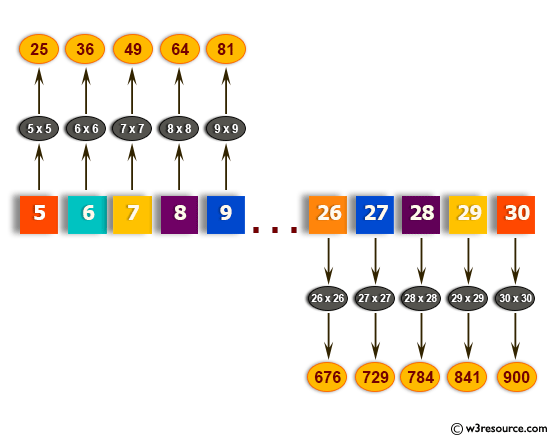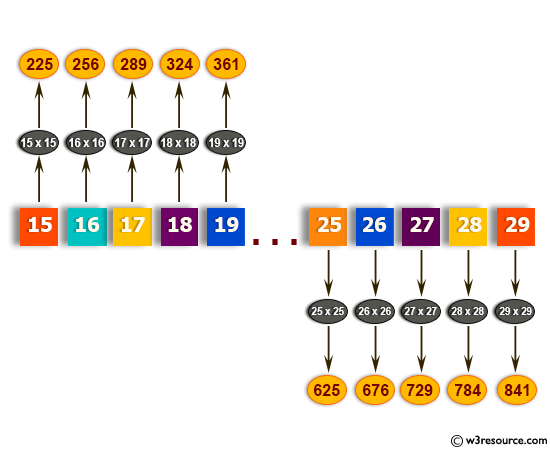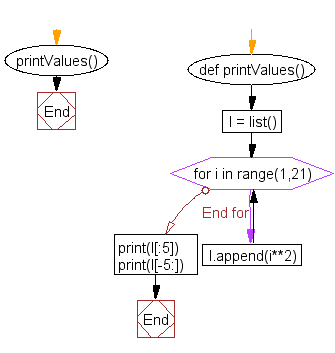﻿ Python: Generate and print a list of first and last 5 elements where the values are square of numbers between two numbers - w3resourcePython: Generate and print a list of first and last 5 elements where the values are square of numbers between two numbers

Python List: Exercise - 16 with Solution

Write a Python program to generate and print a list of first and last 5 elements where the values are square of numbers between 1 and 30 (both included).

Example - 1 :Example - 2 :Example - 3 :Sample Solution:-

Python Code:

def printValues():
l = list()
for i in range(1,21):
l.append(i**2)
print(l[:5])
print(l[-5:])

printValues()

Sample Output:

[1, 4, 9, 16, 25]
[256, 289, 324, 361, 400

Flowchart:Visualize Python code execution:

The following tool visualize what the computer is doing step-by-step as it executes the said program:

Python Code Editor:

Have another way to solve this solution? Contribute your code (and comments) through Disqus.

What is the difficulty level of this exercise?

﻿